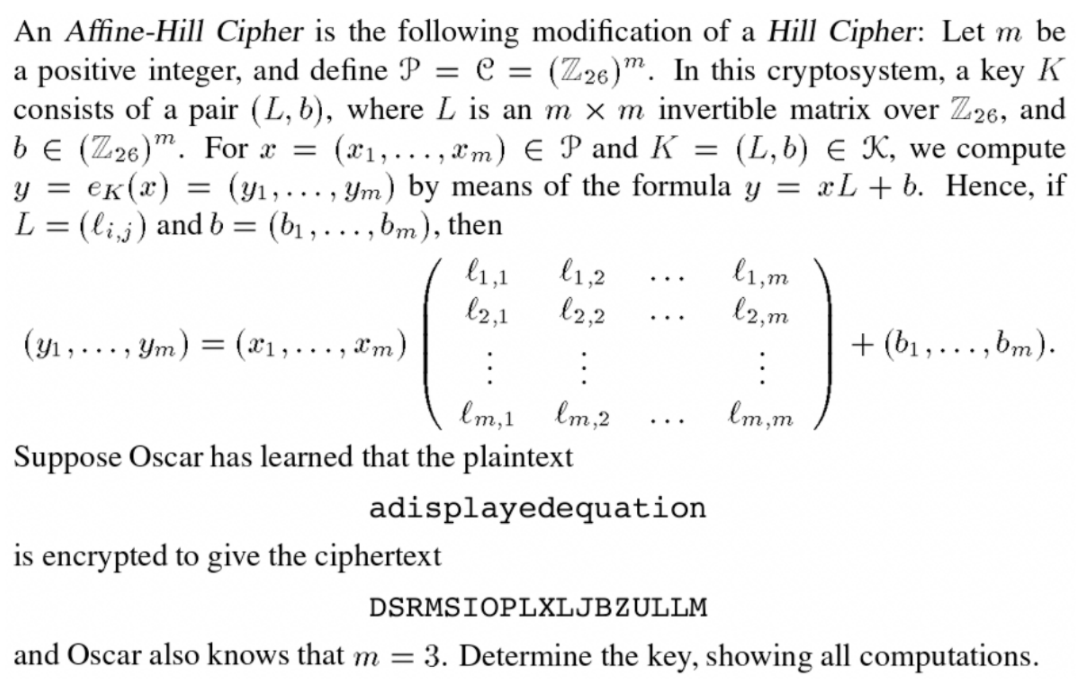# Question Solved1 AnswerAn Affine-Hill Cipher is the following modification of a Hill Cipher: Let m be a positive integer, and define P = C = (Z26)". In this cryptosystem, a key K consists of a pair (L, b), where L is an m x m invertible matrix over Z26, and be (Z26)". For x = (21, ..., Xm) E P and K = (L,b) E K, we compute y = ek(x) = (y1, ... , Ym) by means of the formula y = xL + b. Hence, if L = (li,j) and b = (bi, ...,bm), then l1, m 11,2 11,1 12,1 12,2 l2, m (41, ... , Ym) = (x1, ..., 8m) + (61, ...,bm). : : : lm, 1 lm, 2 Im,m Suppose Oscar has learned that the plaintext adisplayedequation is encrypted to give the ciphertext DSRMSIOPLXLJBZULLM and Oscar also knows that m = 3. Determine the key, showing all computations.Transcribed Image Text: An Affine-Hill Cipher is the following modification of a Hill Cipher: Let m be a positive integer, and define P = C = (Z26)". In this cryptosystem, a key K consists of a pair (L, b), where L is an m x m invertible matrix over Z26, and be (Z26)". For x = (21, ..., Xm) E P and K = (L,b) E K, we compute y = ek(x) = (y1, ... , Ym) by means of the formula y = xL + b. Hence, if L = (li,j) and b = (bi, ...,bm), then l1, m 11,2 11,1 12,1 12,2 l2, m (41, ... , Ym) = (x1, ..., 8m) + (61, ...,bm). : : : lm, 1 lm, 2 Im,m Suppose Oscar has learned that the plaintext adisplayedequation is encrypted to give the ciphertext DSRMSIOPLXLJBZULLM and Oscar also knows that m = 3. Determine the key, showing all computations.
More
Transcribed Image Text: An Affine-Hill Cipher is the following modification of a Hill Cipher: Let m be a positive integer, and define P = C = (Z26)". In this cryptosystem, a key K consists of a pair (L, b), where L is an m x m invertible matrix over Z26, and be (Z26)". For x = (21, ..., Xm) E P and K = (L,b) E K, we compute y = ek(x) = (y1, ... , Ym) by means of the formula y = xL + b. Hence, if L = (li,j) and b = (bi, ...,bm), then l1, m 11,2 11,1 12,1 12,2 l2, m (41, ... , Ym) = (x1, ..., 8m) + (61, ...,bm). : : : lm, 1 lm, 2 Im,m Suppose Oscar has learned that the plaintext adisplayedequation is encrypted to give the ciphertext DSRMSIOPLXLJBZULLM and Oscar also knows that m = 3. Determine the key, showing all computations.